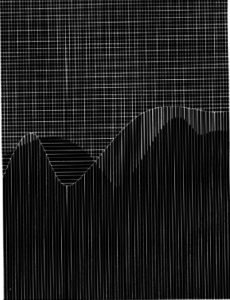# Computer Graphics and Art Rendition

When I first started recreating “Phase Pattern” by Manfred Mohr, I considered using functions like line() and beginShape(), but then I thought that drawing graphs would add more accuracy to the patterns I was trying to create. So I drew some sine and cosine functions, added some vertical and horizontal lines here and there, and voila! Can’t say it’s my best work because it still needs some adjustments with the curve shape, but I’ll keep working on it with my not so extensive math skills.

“Phase Patterns” by Mohr:And here’s my code:

```float theta = 0.0;  // Start angle at 0
float frequency = 2.0;
float amplitude = 50.0;  // Height of wave
float period = 500.0;  // How many pixels before the wave repeats

float y_for_sine(float x){
float y = sin(x * TWO_PI * frequency/width) * 100;
y = y + height/2 + 50;
return y;
}

float y_for_cos(float x){
float y = cos(x * TWO_PI * frequency/width) * 100;
y = y + height/2;
return y;
}

void setup() {
size(600, 700);
background(0);
}

void draw(){
// bottom vertical lines (cos)
stroke(255);
float strokeweight2 = 1.0;
strokeWeight(strokeweight2);
//FOR EACH CURVE:
float interval2 = (int) strokeweight2 + 4.89;
float x_index2 = 0;
while(x_index2 < width){
//line(x_index, 0, x_index, height);
for(int y = 0; y < height; y++){
//draw a point at (x,y) if y < y_for_sine(y)
if(y > y_for_cos(x_index2)){
point(x_index2,y );
}
}
x_index2 += interval2;
}

//top horizental lines (cos)
float strokeweight3 = 1.0;
strokeWeight(strokeweight2);
//FOR EACH CURVE:
float interval3 = (int) strokeweight2 + 6;
float x_index3 = 0;
while(x_index3 < width){
//line(x_index, 0, x_index, height);
for(int y = 0; y < height; y += interval3){
//draw a point at (x,y) if y < y_for_sine(y)
if(y < y_for_cos(x_index3)){
point(x_index3,y );
}
}
x_index3 += 1;
}

//top vertical lines (sin)
stroke(255);
float strokeweight = 1.0;
strokeWeight(strokeweight);
//FOR EACH CURVE:
float interval= (int) strokeweight + 5.054;
float x_index = 0;
while(x_index < width){
//line(x_index, 0, x_index, height);
for(int y = 0; y < height; y++){
//draw a point at (x,y) if y < y_for_sine(y)
if(y < y_for_sine(x_index)){
point(x_index,y);
}
}
x_index += interval;
}

// cos line -- make this thicker
strokeWeight(1.05);
for(int x = 0; x < width; x++){
point(x, y_for_cos(x));
}
}
```